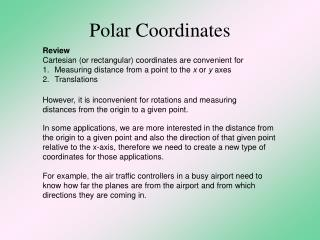# Polar Coordinates - PowerPoint PPT PresentationDownload PresentationPolar Coordinates

Polar Coordinates
Download Presentation## Polar Coordinates

- - - - - - - - - - - - - - - - - - - - - - - - - - - E N D - - - - - - - - - - - - - - - - - - - - - - - - - - -
##### Presentation Transcript

1. Polar Coordinates • Review • Cartesian (or rectangular) coordinates are convenient for • Measuring distance from a point to the x or y axes • Translations However, it is inconvenient for rotations and measuring distances from the origin to a given point. In some applications, we are more interested in the distance from the origin to a given point and also the direction of that given point relative to the x-axis, therefore we need to create a new type of coordinates for those applications. For example, the air traffic controllers in a busy airport need to know how far the planes are from the airport and from which directions they are coming in.

2. Conversion from Polar to Cartesian Conversion from Cartesian to Polar

3. Disadvantages of the Polar Coordinates 1. The coordinates of a given point is never unique because the radius can be positive or negative, and the angle θ can be bigger than 360 2. At the Pole, the angle θ is undefined.

4. Polar Curves In polar coordinates, it is usually more convenient to express the radius r as a function of the angle , i.e. r = f () • Example: • r = 5 will produce a circle of radius 5 centered at the origin. • r =  will produce a spiral starting at the Pole.

5. Polar Curve r = 0.5 + cosθ

6. Polar Curve r = 0.5 + cosθ

7. Polar Curve r = 0.5 + cosθ

8. Polar Curve r = 0.5 + cosθ

9. Polar Curve r = 0.5 + cosθ

10. Polar Curve r = 0.5 + cosθ

11. Polar Curve r = 0.5 + cosθ

12. Polar Curve r = 0.5 + cosθ

13. Polar Curve r = 0.5 + cosθ

14. Polar Curve r = 0.5 + cosθ

15. Polar Curve r = 0.5 + cosθ

16. Polar Curve r = 0.5 + cosθ

17. Polar Curve r = 0.5 + cosθ

18. Polar Curve r = 0.5 + cosθ

19. Polar Curve r = 0.5 + cosθ

20. Polar Curve r = 0.5 + cosθ

21. Polar Curve r = 0.5 + cosθ

22. Polar Curve r = 0.5 + cosθ

23. Polar Curve r = 0.5 + cosθ

24. Polar Curve r = 0.5 + cosθ

25. Polar Curve r = 0.5 + cosθ

26. Polar Curve r = 0.5 + cosθ

27. Polar Curve r = 0.5 + cosθ

28. Polar Curve r = 0.5 + cosθ

29. Polar Curve r = 0.5 + cosθ

30. Polar Curve r = 0.5 + cosθ

31. Polar Curve r = 0.5 + cosθ

32. Polar Curve r = 0.5 + cosθ

33. Polar Curve r = 0.5 + cosθ

34. Polar Curve r = 0.5 + cosθ

35. Polar Curve r = 0.5 + cosθ

36. Polar Curve r = 0.5 + cosθ

37. Polar Curve r = 0.5 + cosθ

38. Polar Curve r = 0.5 + cosθ